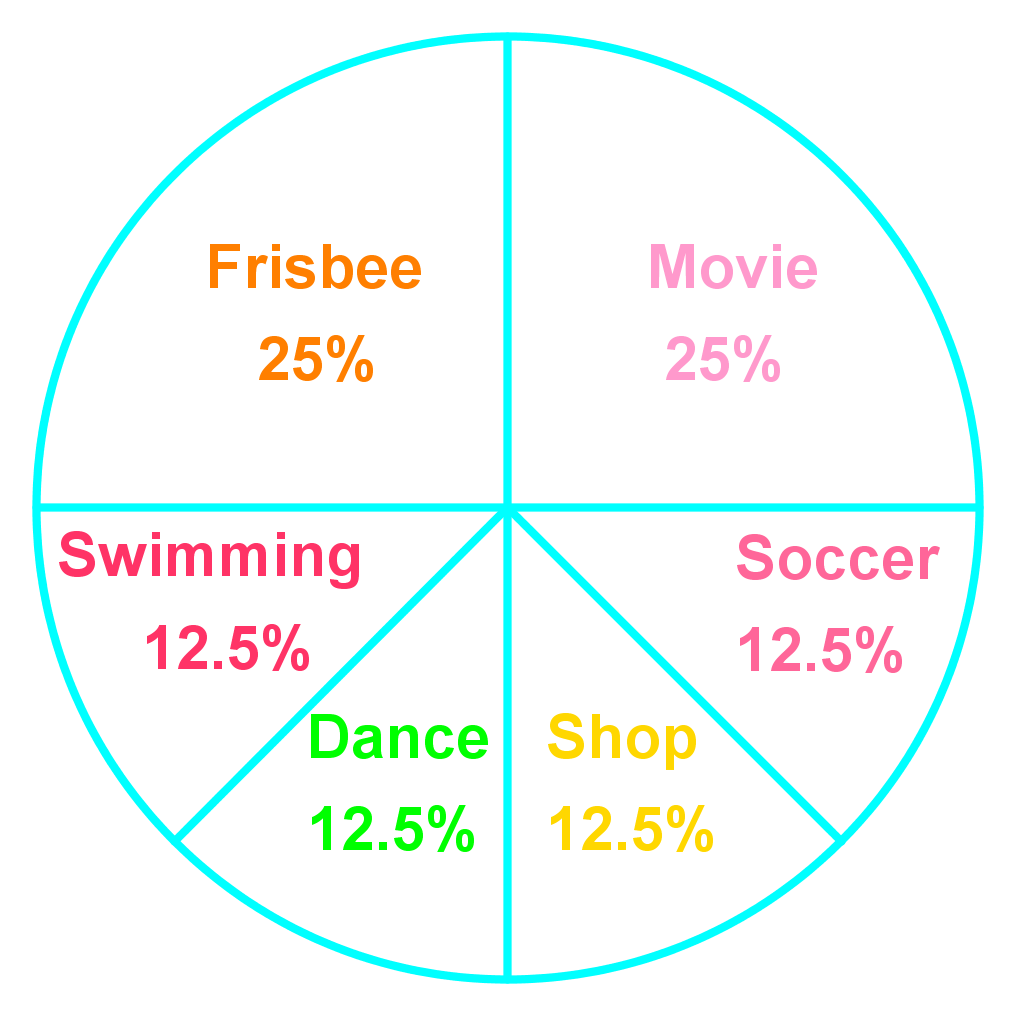# Introduction to probability

Practice Now
0/8

### Examples

#### Lessons

1. Below is a probability model showing the weathers in fall in City A.
 Weather Probability Rain 0.35 Snow 0.08 Sunny 0.16 Cloudy 0.41

1. What is P(snow)? Write your answer as a fraction, a ratio and a percent.
2. Is it more likely to be sunny than cloudy in fall in City A?
2. Each student in a class of 30 writes his or her name on a slip of paper. These slips of paper are folded and put in a jar. Half of these slips are blue and half are yellow.
1. What is the probability that a blue slip will be picked? Write your answer as a fraction, a decimal, a ratio, and a percent.
2. Rob, Jennie and Alice are in this class. What is the probability that one of these students' names will be picked? Write your answer as a fraction, a decimal, a ratio, and a percent.
3. Jen and her friend spin the wheel to decide what fun activity they will do this afternoon.1. What is the probability that the spin will land on movie or swimming? Write your answer as a percent and decimal.
2. What is the probability that the spin will not land on dancing or shopping? Write your answer as a percent and fraction.
3. If they spin the wheel twice, what is the probability that the spin will land on soccer and frisbee? Write your answer as a percent and fraction.
0%

## Become a Member to Get More!

• #### Easily See Your ProgressWe track the progress you've made on a topic so you know what you've done. From the course view you can easily see what topics have what and the progress you've made on them. Fill the rings to completely master that section or mouse over the icon to see more details.

• #### Make Use of Our Learning Aids###### Practice Accuracy

See how well your practice sessions are going over time.

Stay on track with our daily recommendations.

• #### Earn Achievements as You LearnMake the most of your time as you use StudyPug to help you achieve your goals. Earn fun little badges the more you watch, practice, and use our service.

• #### Create and Customize Your AvatarPlay with our fun little avatar builder to create and customize your own avatar on StudyPug. Choose your face, eye colour, hair colour and style, and background. Unlock more options the more you use StudyPug.

###### Topic Basics
In this section, we practice finding the probability of events. Probability is the likelihood or chance of an event occurring. We will practice expressing probabilities as ratios, fractions, decimals, and percents. Probability can be calculated using the following equation: $Probability=\frac{Favourable\ outcomes}{ Possible\ outcomes}$. P(blue) is a short way to write "the probability of blue".